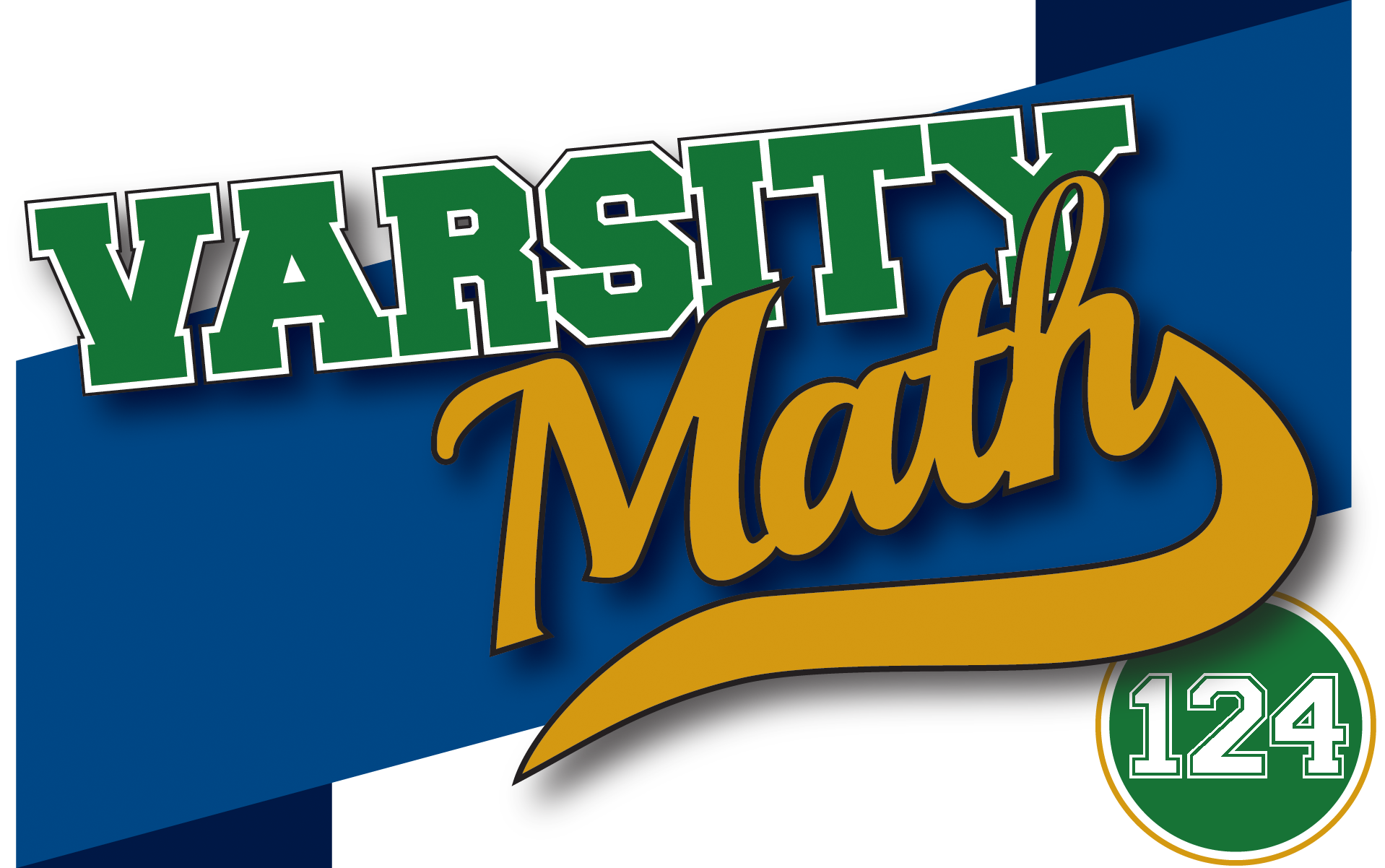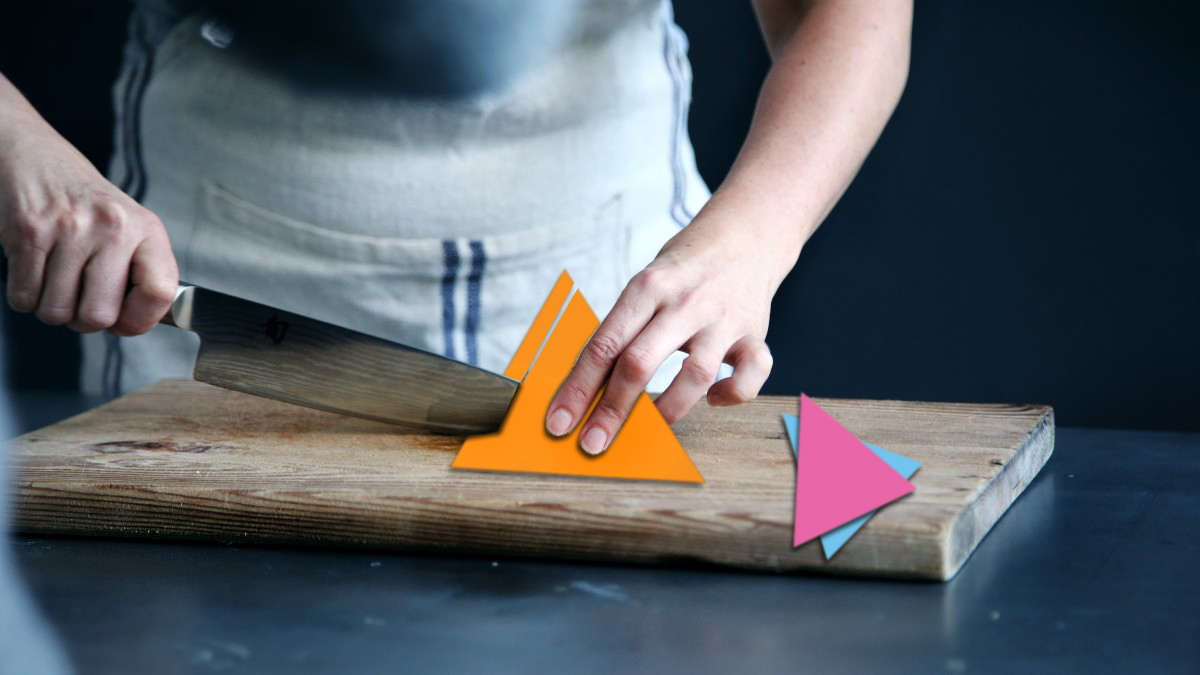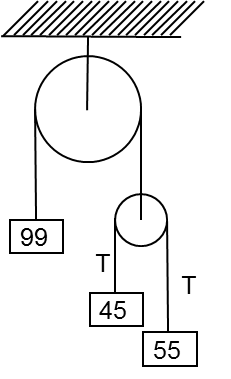## ________________

This week, the coach has two challenges to hone the team’s geometric and visualization skills.

## ________________### Cutting the Triangle

Cut an equilateral triangle into three pieces of identical shape where:

(a) They are all the same size.
(b) They are all of different sizes.
(c) Two are the same size and the third is a different size.

Pieces may be turned over.

### Square Repair

A square has had a 45°-30°-105° triangle cut from it and pasted to its right edge, as seen in the figure below.

Cut the figure into two pieces in a different way that will still allow assembly into the original square.## Solutions to week 123

In Mining Mishap, the miner was 66 inches up the ladder. In Pulley Puzzle, the 99-weight will stay at rest upon release. The 45-weight accelerates upward at g/10 and the 55-weight accelerates downward at g/10, where g is the acceleration due to gravity.

Mining Mishap answer explained:
If the rock falls from a distance d above the top of the ladder, it takes a time of √(2d/g) seconds to reach the top of the ladder. If the miner is h feet up the ladder and climbs at 4 feet per second then he would take (10 – h)/4 seconds to reach the top of the ladder. Thus, 2d/g = (10 – h)2/16. The rock takes √[(2d + 20)/g] seconds to reach the bottom of the ladder and the miner would take h/4 seconds to reach the bottom of the ladder. Thus, (2d + 20)/g = h2/16. If we take the difference of the two equations, we get 20/g = 10(2h – 10)/16, which leads to h = 5.5 feet. Thus, the miner was 66 inches up the ladder.

Pulley Puzzle answer explained:
If the 99-weight remains stationary then the tension in the rope around the larger wheel must be 99g and the tension in the rope around the smaller wheel must be T = 99g/2, where g is the acceleration due to gravity. The upward acceleration of the 45-weight must equal the downward acceleration of the 55-weight and, indeed, a45 = T/45 – g = 11g/10 – g = g/10 and a55 = g – T/55 = g – 9g/10 = g/10.## Recent Weeks

Links to all of the puzzles and solutions are on the Complete Varsity Math page.

Come back next week for answers and more puzzles.